# RS Aggarwal Solutions for Class 10 Maths Chapter 17 Volume and Surface Area of Solids Exercise 17B

R S Aggarwal Solutions for Class 10 Maths Chapter 17 Volume and Surface Area of Solids exercise involving the conversion of solid from one shape to another and mixed problems. We provide stepwise answers to all the questions from the chapters enlisted in the textbook. R S Aggarwal Maths Chapter 17 study material for class 10 is very useful for students as it helps them to score well in the class 10 exams.

## Download PDF of RS Aggarwal Solutions for Class 10 Maths Chapter 17 Volume and Surface Area of Solids Exercise 17B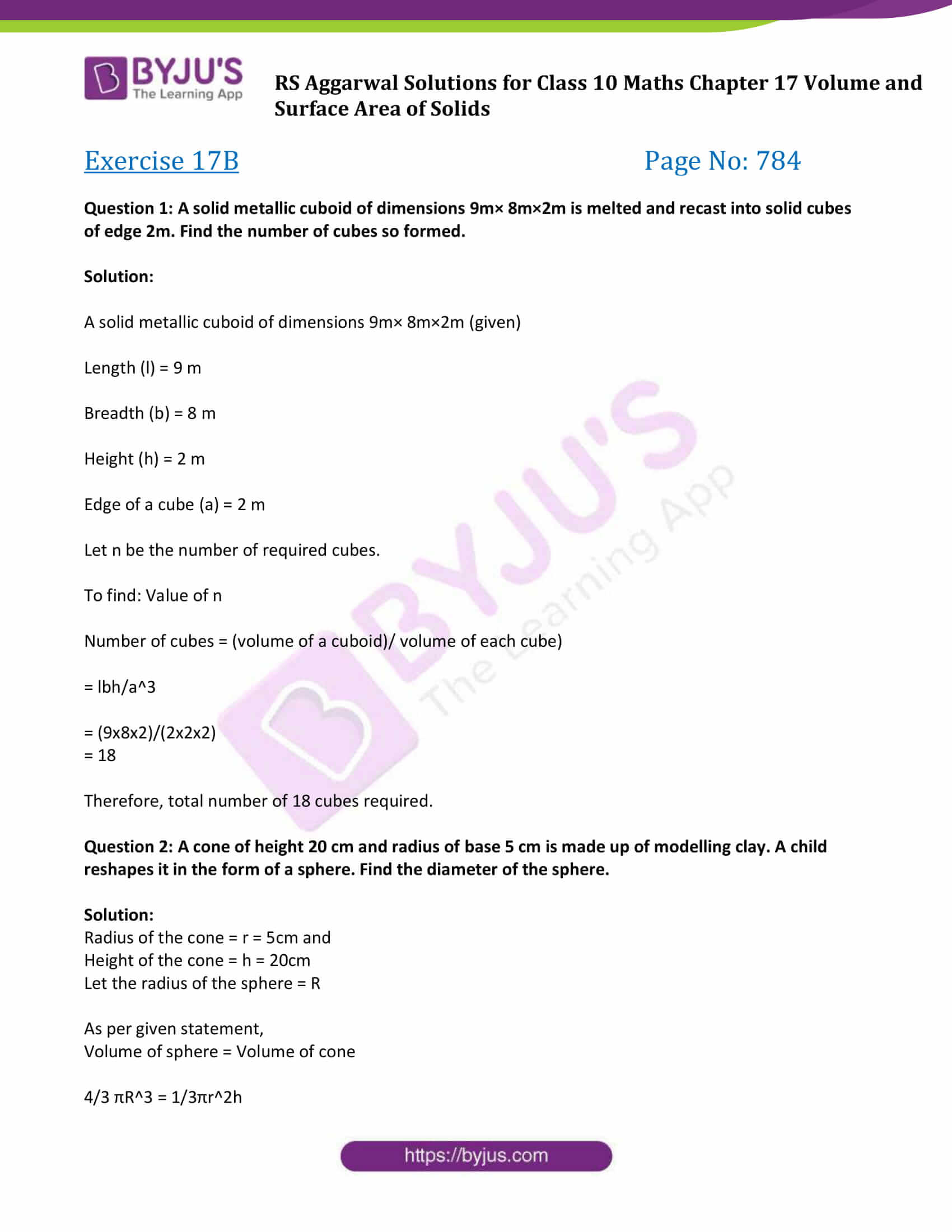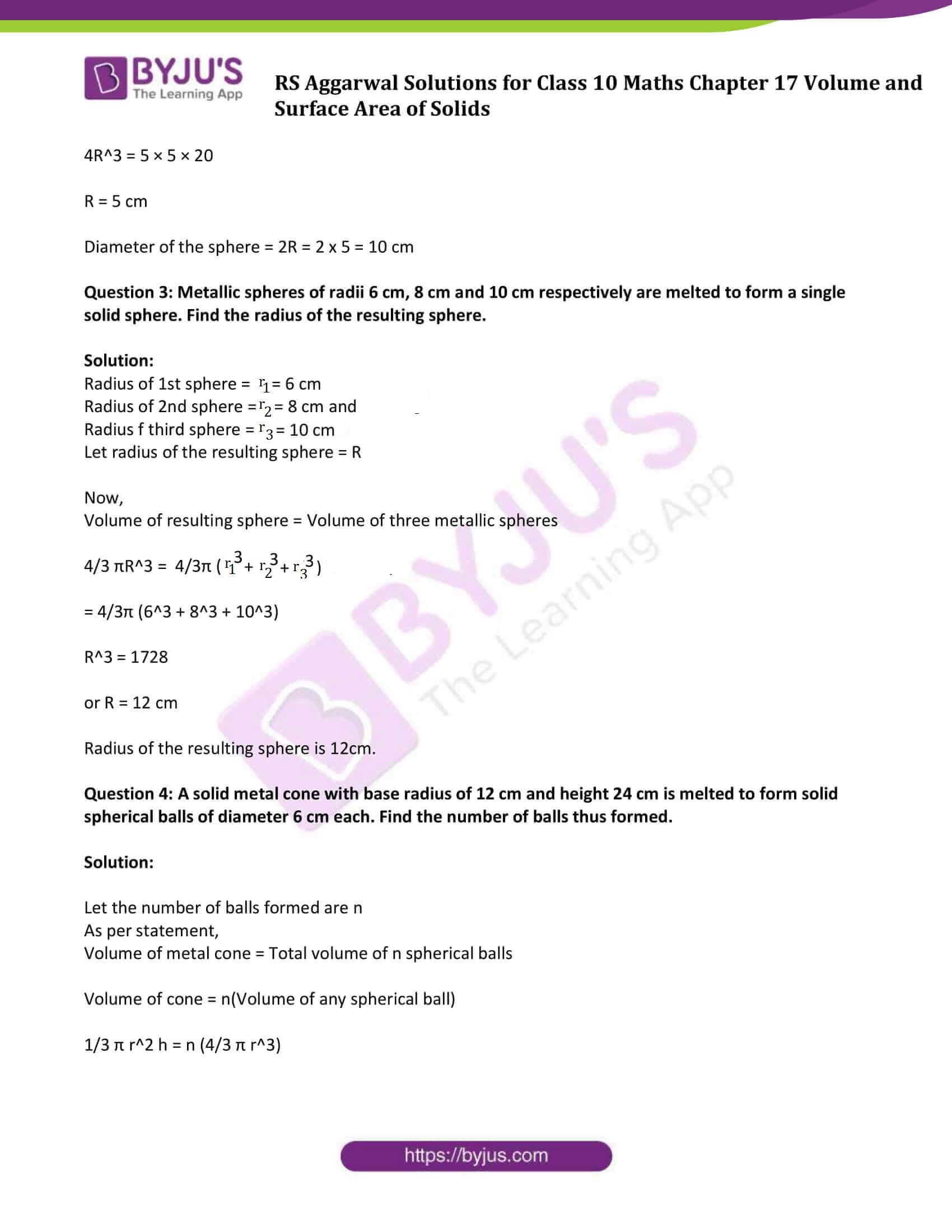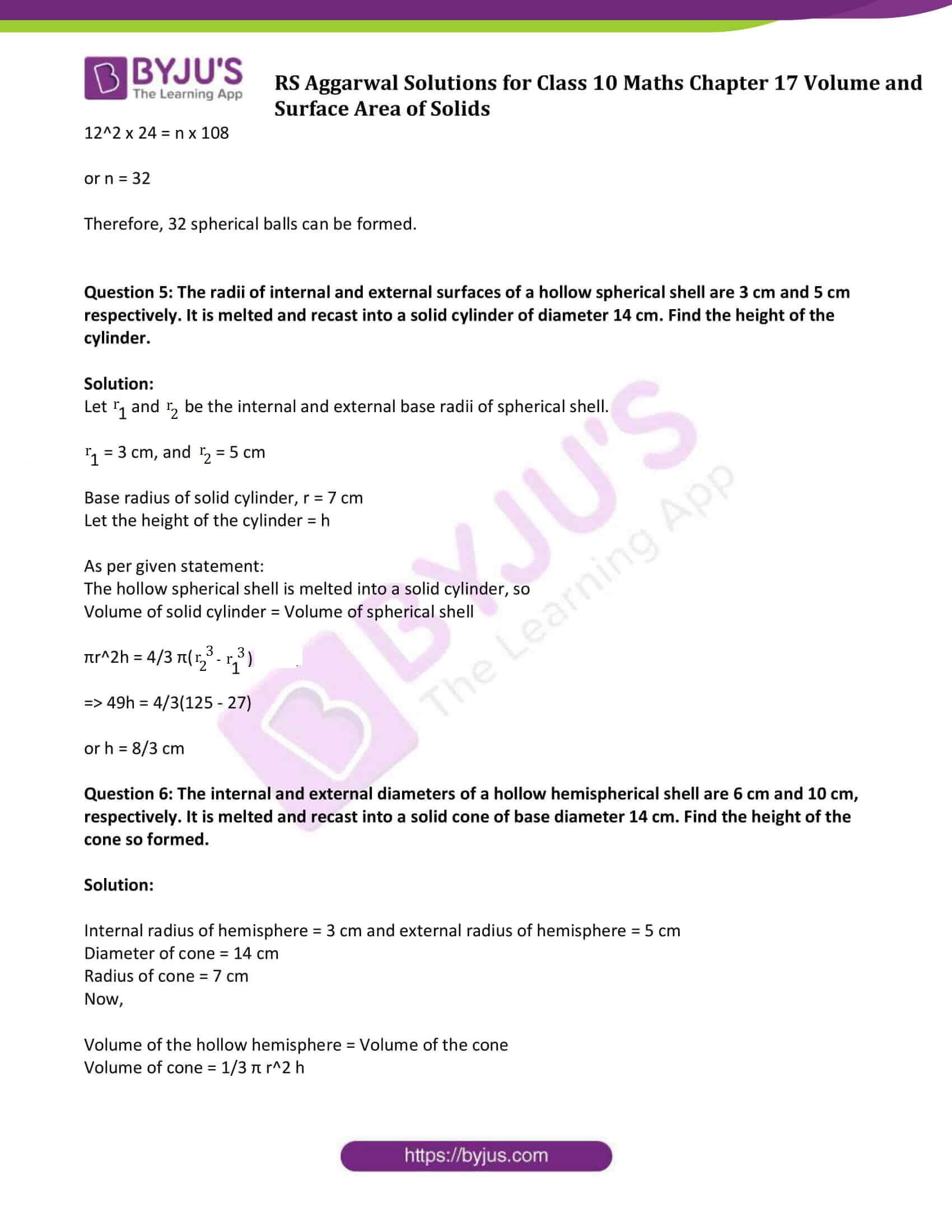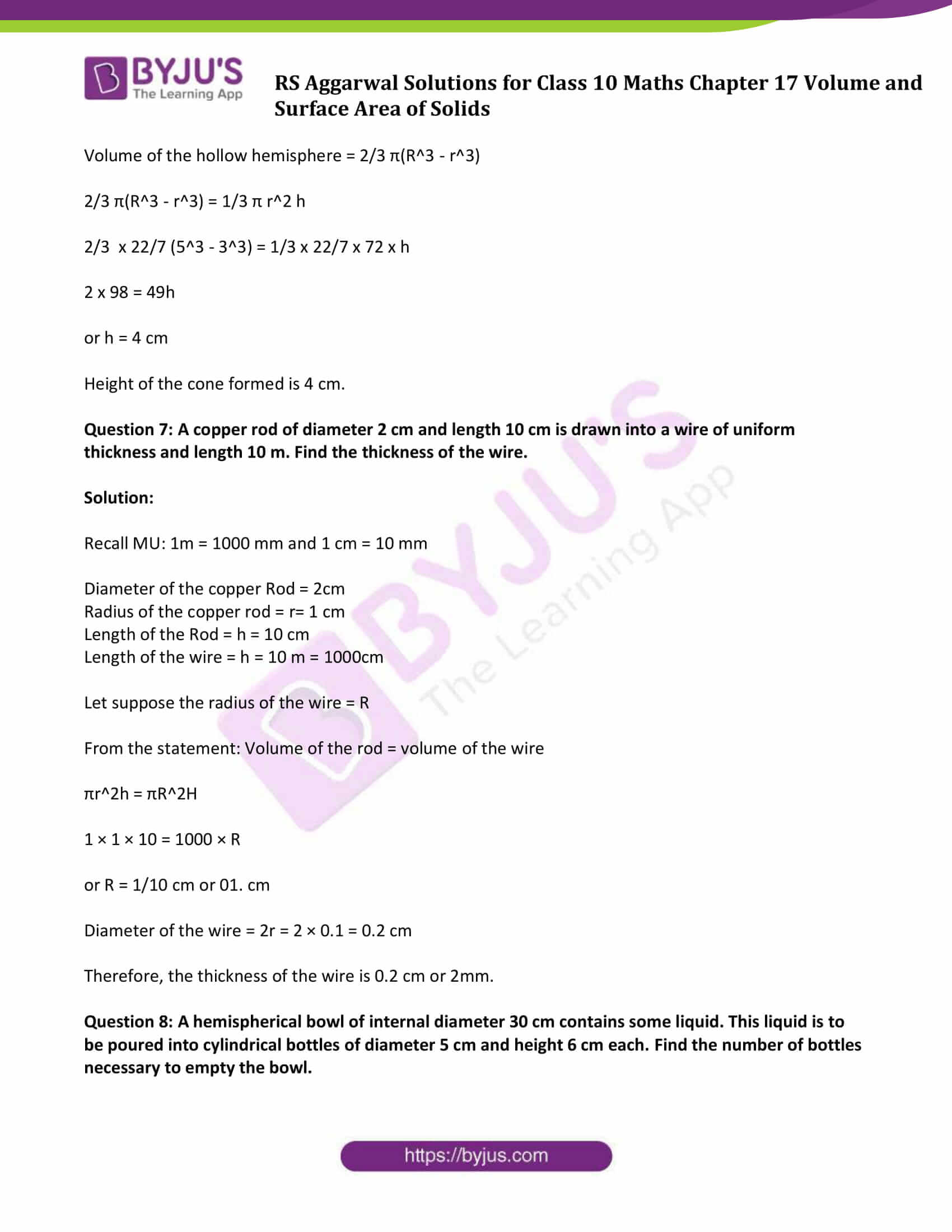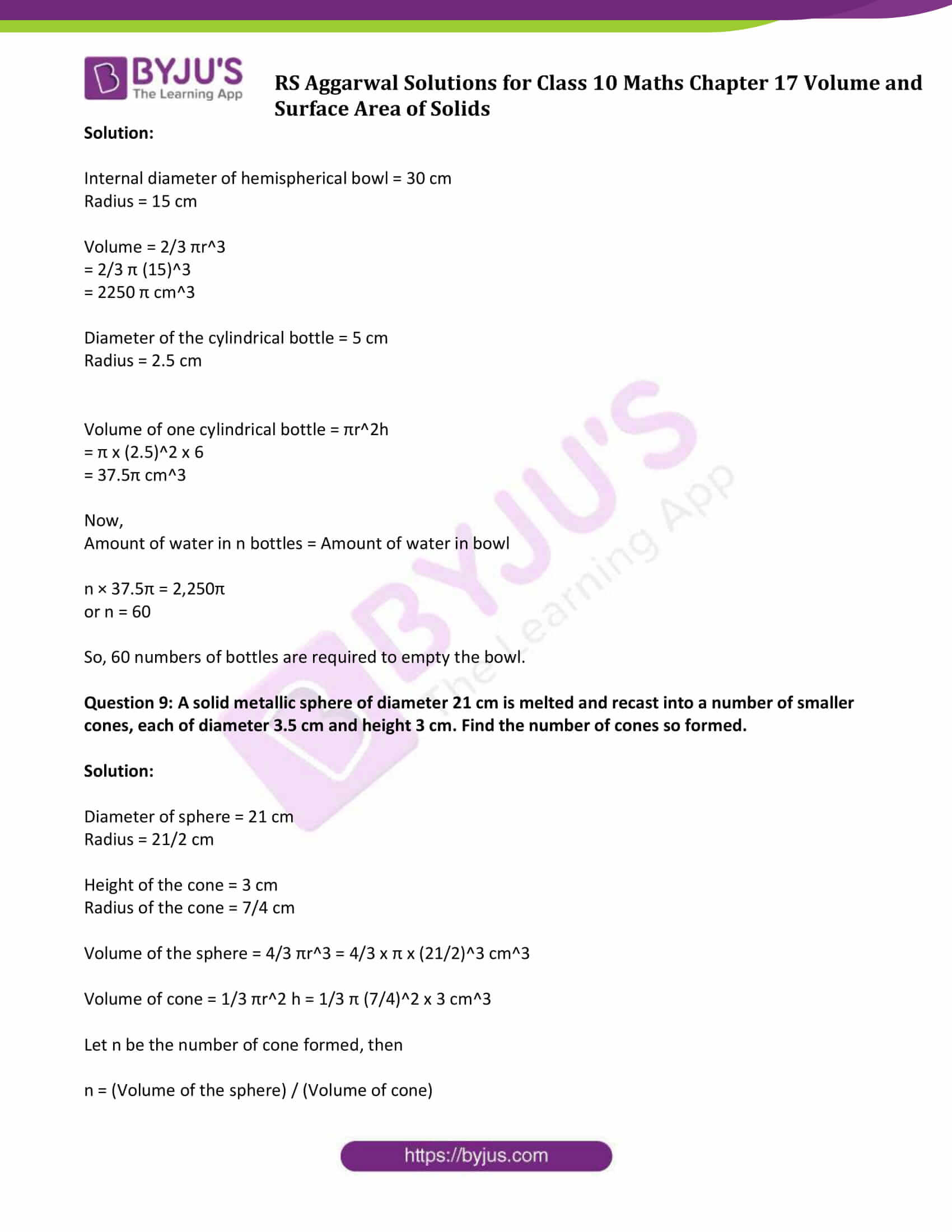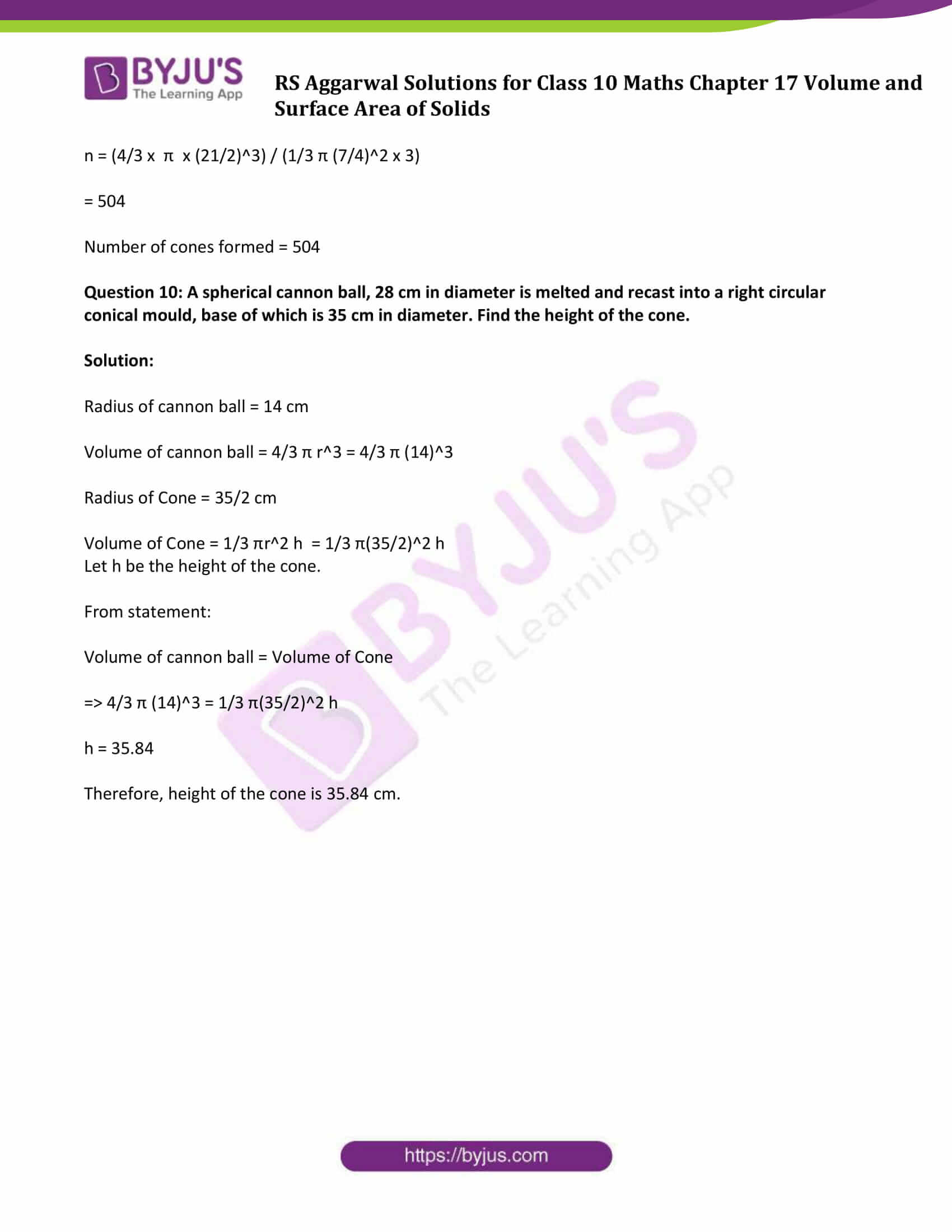### Access other exercise solutions of Class 10 Maths Chapter 17 Volume and Surface Area of Solids

Exercise 17 A Solutions

Exercise 17 C Solutions

Exercise 17 D Solutions

### Access Solutions of Maths RS Aggarwal Class 10 Chapter 17 – Volume and Surface Area of Solids Exercise 17B

Question 1: A solid metallic cuboid of dimensions 9m× 8m×2m is melted and recast into solid cubes of edge 2m. Find the number of cubes so formed.

Solution:

A solid metallic cuboid of dimensions 9m× 8m×2m (given)

Length (l) = 9 m

Height (h) = 2 m

Edge of a cube (a) = 2 m

Let n be the number of required cubes.

To find: Value of n

Number of cubes = (volume of a cuboid)/ volume of each cube)

= lbh/a3

= (9x8x2)/(2x2x2)

= 18

Therefore, total number of 18 cubes required.

Question 2: A cone of height 20 cm and radius of base 5 cm is made up of modelling clay. A child reshapes it in the form of a sphere. Find the diameter of the sphere.

Solution:

Radius of the cone = r = 5cm and

Height of the cone = h = 20cm

Let the radius of the sphere = R

As per given statement,

Volume of sphere = Volume of cone

4/3 πR3 = 1/3πr2h

4R3 = 5 × 5 × 20

R = 5 cm

Diameter of the sphere = 2R = 2 x 5 = 10 cm

Question 3: Metallic spheres of radii 6 cm, 8 cm and 10 cm respectively are melted to form a single solid sphere. Find the radius of the resulting sphere.

Solution:

Radius of 1st sphere = r1 = 6 cm

Radius of 2nd sphere =r2 = 8 cm and

Radius f third sphere = r3 = 10 cm

Let radius of the resulting sphere = R

Now,

Volume of resulting sphere = Volume of three metallic spheres

4/3 πR3 = 4/3π (r13 + r23 + r33)

= 4/3π (63 + 83 + 103)

R3 = 1728

or R = 12 cm

Radius of the resulting sphere is 12cm.

Question 4: A solid metal cone with base radius of 12 cm and height 24 cm is melted to form solid spherical balls of diameter 6 cm each. Find the number of balls thus formed.

Solution:

Let the number of balls formed are n

As per statement,

Volume of metal cone = Total volume of n spherical balls

Volume of cone = n(Volume of any spherical ball)

1/3 π r2 h = n (4/3 π r3)

122 x 24 = n x 108

or n = 32

Therefore, 32 spherical balls can be formed.

Question 5: The radii of internal and external surfaces of a hollow spherical shell are 3 cm and 5 cm respectively. It is melted and recast into a solid cylinder of diameter 14 cm. Find the height of the cylinder.

Solution:

Let r1 and r2 be the internal and external base radii of spherical shell.

r1 = 3 cm, and r2 = 5 cm

Base radius of solid cylinder, r = 7 cm

Let the height of the cylinder = h

As per given statement:

The hollow spherical shell is melted into a solid cylinder, so

Volume of solid cylinder = Volume of spherical shell

πr2h = 4/3 π(r23 – r13)

⇨ 49h = 4/3(125 – 27)

or h = 8/3 cm

Question 6: The internal and external diameters of a hollow hemispherical shell are 6 cm and 10 cm, respectively. It is melted and recast into a solid cone of base diameter 14 cm. Find the height of the cone so formed.

Solution:

Internal radius of hemisphere = 3 cm and external radius of hemisphere = 5 cm

Diameter of cone = 14 cm

Radius of cone = 7 cm

Now,

Volume of the hollow hemisphere = Volume of the cone

Volume of cone = 1/3 π r2 h

Volume of the hollow hemisphere = 2/3 π(R3 – r3)

2/3 π(R3 – r3) = 1/3 π r2 h

2/3 x 22/7 (53 – 33) = 1/3 x 22/7 x 72 x h

2 x 98 = 49h

or h = 4 cm

Height of the cone formed is 4 cm.

Question 7: A copper rod of diameter 2 cm and length 10 cm is drawn into a wire of uniform thickness and length 10 m. Find the thickness of the wire.

Solution:

Recall MU: 1m = 1000 mm and 1 cm = 10 mm

Diameter of the copper Rod = 2cm

Radius of the copper rod = r= 1 cm

Length of the Rod = h = 10 cm

Length of the wire = h = 10 m = 1000cm

Let suppose the radius of the wire = R

From the statement: Volume of the rod = volume of the wire

πr2h = πR2H

1 × 1 × 10 = 1000 × R

or R = 1/10 cm or 01. cm

Diameter of the wire = 2r = 2 × 0.1 = 0.2 cm

Therefore, the thickness of the wire is 0.2 cm or 2mm.

Question 8: A hemispherical bowl of internal diameter 30 cm contains some liquid. This liquid is to be poured into cylindrical bottles of diameter 5 cm and height 6 cm each. Find the number of bottles necessary to empty the bowl.

Solution:

Internal diameter of hemispherical bowl = 30 cm

Volume = 2/3 πr3

= 2/3 π (15)3

= 2250 π cm3

Diameter of the cylindrical bottle = 5 cm

Volume of one cylindrical bottle = πr2h

= π x (2.5)2 x 6

= 37.5π cm3

Now,

Amount of water in n bottles = Amount of water in bowl

n × 37.5π = 2,250π

or n = 60

So, 60 numbers of bottles are required to empty the bowl.

Question 9: A solid metallic sphere of diameter 21 cm is melted and recast into a number of smaller cones, each of diameter 3.5 cm and height 3 cm. Find the number of cones so formed.

Solution:

Diameter of sphere = 21 cm

Height of the cone = 3 cm

Radius of the cone = 7/4 cm

Volume of the sphere = 4/3 πr3 = 4/3 x π x (21/2)3 cm3

Volume of cone = 1/3 πr2 h = 1/3 π (7/4)2 x 3 cm3

Let n be the number of cone formed, then

n = (Volume of the sphere) / (Volume of cone)

n = (4/3 x π x (21/2)3) / (1/3 π (7/4)2 x 3)

= 504

Number of cones formed = 504

Question 10: A spherical cannon ball, 28 cm in diameter is melted and recast into a right circular conical mould, base of which is 35 cm in diameter. Find the height of the cone.

Solution:

Radius of cannon ball = 14 cm

Volume of cannon ball = 4/3 π r3 = 4/3 π (14)3

Radius of Cone = 35/2 cm

Volume of Cone = 1/3 πr2 h = 1/3 π(35/2)2 h

Let h be the height of the cone.

From statement:

Volume of cannon ball = Volume of Cone

⇨ 4/3 π (14)3 = 1/3 π(35/2)2 h

h = 35.84

Therefore, height of the cone is 35.84 cm.

## RS Aggarwal Solutions for Class 10 Maths Chapter 17 Volume and Surface Area of Solids Exercise 17B Topics:

Class 10 Maths Chapter 17 Volume and Surface Area of Solids Exercise 17B is based on the below topic:

• Conversion of solid from one shape to another and mixed problems